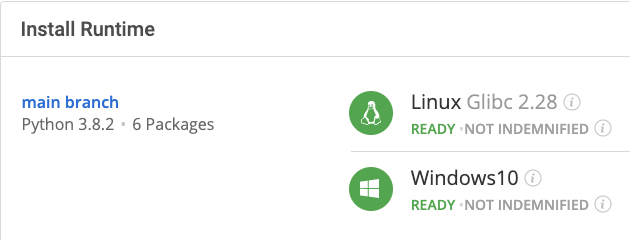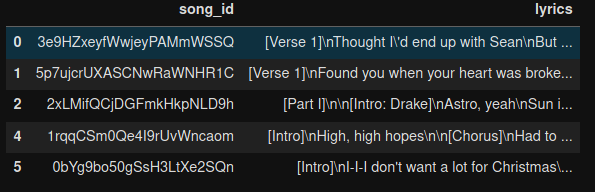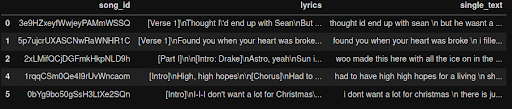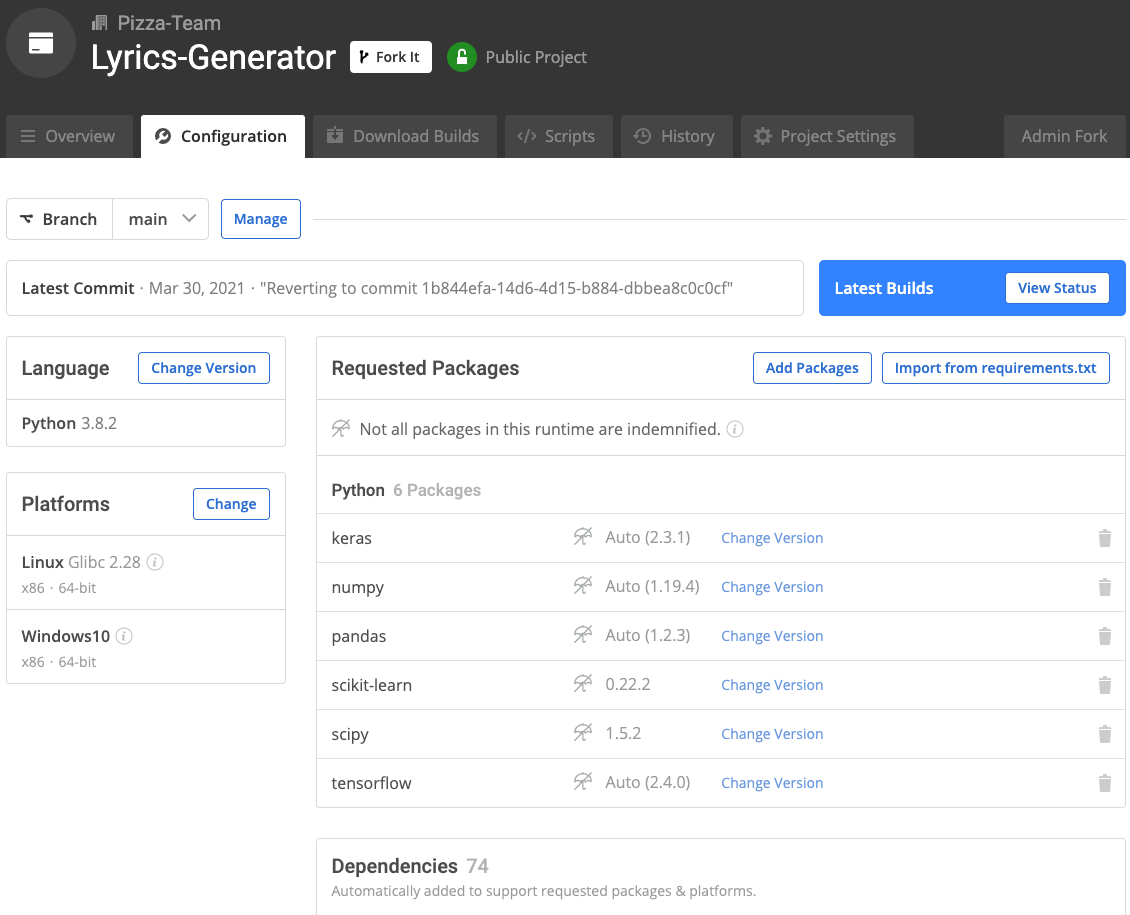Last Updated: December 24, 2021

# How to Build a Lyrics Generator with Python & Recurrent Neural Networks

Text generation is one of the most common examples of applied Machine Learning (ML). The constant evolution of algorithms and the huge amount of text data available allows us to train models that try to capture context or imitate previous work from others. In this way, ML has been used to do everything from writing journalistic articles to short stories to entire novels. Unfortunately, many of them are obviously poor examples of writing mainly because the ML routines often generate nonsensical sentences and generate long form documents with poor structural coherence.

Interestingly, these shortcomings actually work in ML’s favor when it comes to writing poetry, and by corollary, lyrics since they’re more focused on generating an emotional response rather than an intellectual one in the reader/listener. Given a set of input keywords, an ML-driven lyric generator will produce a poem that won’t win any awards, but will likely evoke a response from the reader, even if it’s just to laugh at its absurdity.

In this article we are going to use ML to build a routine that will automatically generate lyrics for us. To do so, we’ll follow a set of steps:

2. Cleanse and combine the data
3. Create a Recurrent Neural Network (RNN)
4. Evaluate the results

## Before you start: Install Our Lyrics Generator Ready-To-Use Python Environment

The easiest way to get started building your model is to install our Lyrics Generator Python environment for Windows or Linux, which contains a version of Python and all of the packages you need.Or you could also use our State tool to install this runtime environment.

For Windows users, run the following at a CMD prompt to automatically download and install our CLI, the State Tool along with the Lyrics Generator runtime into a virtual environment:

`powershell -Command "& \$([scriptblock]::Create((New-Object Net.WebClient).DownloadString('https://platform.activestate.com/dl/cli/install.ps1'))) -activate-default Pizza-Team/Lyrics-Generator"`

For Linux users, run the following to automatically download and install our CLI, the State Tool along with the Lyrics Generator runtime into a virtual environment:

`sh <(curl -q https://platform.activestate.com/dl/cli/install.sh) --activate-default Pizza-Team/Lyrics-Generator`

### 1–Import Lyric and Poetry Data

Ok, first of all we’re going to need to get some training data, which should consist of the lyrics for actual songs.

There are several datasets available, including:

• Million Song Dataset, which includes bags of words, tags and similarity, genres and many other features distributed in several files
• MusicMood dataset, which contains around 10,000 songs labeled for sentiment analysis
• Genius.com, specifically the LyricsGenius package

But as every data engineer knows, the best code is the code you don’t have to maintain yourself. So, I’m going to choose the MusicOSet dataset, which aggregates data from several sources (including Genius.com) into a CSV.

But to improve the quality of the rhymes our ML routine will generate, we can also add some poems from The Poetry Foundation through Kaggle. This dataset (available as a CSV) contains some 14,000 poems, including the author and, in some cases, tags associated with the poem. The idea is to combine both sources to increase the chances of obtaining some useful verses.

The following code will import our two data sources, and initialize a string translator to clean the punctuation from the text before training:

```import io

import os

import sys

import string

import numpy as np

import pandas as pd

from tensorflow import keras

from __future__ import print_function

from tensorflow.keras.models import Sequential

from sklearn.model_selection import train_test_split

from tensorflow.keras.callbacks import LambdaCallback, ModelCheckpoint, EarlyStopping

from tensorflow.keras.layers import Dense, Dropout, Activation, LSTM, Bidirectional, Embedding

translator = str.maketrans('', '', string.punctuation)

The result for the song lyrics shows some 20,000 available songs:### 2–Cleanse the Data

A quick exploration of the data shows that every song lyric contains some meta-labeling in the form of [tag]text[/tag]. With this in mind we can split the intro, verses and chorus for every song, and select only the first four verses (if they are available) plus the chorus. We can apply a simple function to process every song in order to split each verse and join the resulting text in a single text:

```def split_text(x):

text = x['lyrics']

sections = text.split('\\n\\n')

keys = {'Verse 1': np.nan,'Verse 2':np.nan,'Verse 3':np.nan,'Verse 4':np.nan, 'Chorus':np.nan}

lyrics = str()

single_text = []

res = {}

for s in sections:

key = s[s.find('[') + 1:s.find(']')].strip()

if ':' in key:

key = key[:key.find(':')]

if key in keys:

single_text += [x.lower().replace('(','').replace(')','').translate(translator) for x in s[s.find(']')+1:].split('\\n') if len(x) > 1]

res['single_text'] =  ' \n '.join(single_text)

return pd.Series(res)

df = df.join( df.apply(split_text, axis=1))

The result is a new column with the clean lyrics in a single line of text. We have kept the new line separator \n in order to train the model to start a new line when needed:The function to clean the poems text is simpler, and can be applied in a single line of code:

```pdf['single_text'] = pdf['Poem'].apply(lambda x: ' \n '.join([l.lower().strip().translate(translator) for l in x.splitlines() if len(l)>0]))

The result is a new column with the same name of the lyrics dataframe in lowercase without punctuation. Now, we join the two dataframes into a single one we’ll use to train our model:

```sum_df = pd.DataFrame( df['single_text'] )

sum_df = sum_df.append(pd.DataFrame( pdf['single_text'] ))

sum_df.dropna(inplace=True)```

### 3–Creating a Recurrent Neural Network (RNN)

Recall that neural networks are algorithms specialized in recognizing patterns. A basic neural network consists of:

1. An input layer
2. A learned function based on the examples provided
3. An output layer

The learning process consists of minimizing the difference between the calculated output (ie., the ML-generated lyrics) and the target output (ie., our imported lyric/poetry data) for the same input (ie., the same seeded keywords) in a recurrent way.

In the most basic neural network, the input of those algorithms are fixed size vectors, which are computed to obtain a fixed-size result. For example, with image classification a single input vector (the image) produces a single vector of probabilities (ie., the likelihood that the image is a horse or a dog).

More complex neural networks let you solve problems that require a sequence of vectors as input and/or output. Examples include:

• Image captioning, where input image is a single vector but the output is a sequence of text vectors (ie., the description of the image)
• Sentiment analysis, where the input is a sequence of text vectors but the output is a single vector of probabilities (ie., the likelihood that the review is positive vs negative)
• Text translation, where the input is a sequence of text vectors in some language and output is a sequence of text vectors in another language
• Synced video captioning, where input is a sequence of video frame vectors and output is a sequence of text vectors (ie., captions for each frame of the video).

RNNs provide an internal state that is combined with the input and the learned function to produce a new state. This means that RNNs are stateful and can be used to tackle problems that include sequences of vectors as input. In our case we want to use RNNs to create a language model.

A language model is a probability distribution over a sequence of words. It can be used to get the conditional probability of a word given a sequence of previous words. For example, given the sequence [‘Let’, ‘me’, ‘see’, ‘what’, ‘spring’, ‘is’, ‘like’, ‘on’] the model could calculate the probability of jupiter versus paris as the next word in the sequence.

One approach to creating a lyrics generator is to train an RNN to predict the next word in a sentence based on examples extracted from songs and poems. To take this approach, we will first need to:

• Filter our dataset for common versus uncommon words
• Create a training set with sentences composed of a fixed length of words
```text_as_list = []

frequencies = {}

uncommon_words = set()

MIN_FREQUENCY = 7

MIN_SEQ = 5

BATCH_SIZE =  32

def extract_text(text):

global text_as_list

text_as_list += [w for w in text.split(' ') if w.strip() != '' or w == '\n']

sum_df['single_text'].apply( extract_text )

print('Total words: ', len(text_as_list))

for w in text_as_list:

frequencies[w] = frequencies.get(w, 0) + 1

uncommon_words = set([key for key in frequencies.keys() if frequencies[key] < MIN_FREQUENCY])

words = sorted(set([key for key in frequencies.keys() if frequencies[key] >= MIN_FREQUENCY]))

num_words = len(words)

word_indices = dict((w, i) for i, w in enumerate(words))

indices_word = dict((i, w) for i, w in enumerate(words))

print('Words with less than {} appearances: {}'.format( MIN_FREQUENCY, len(uncommon_words)))

print('Words with more than {} appearances: {}'.format( MIN_FREQUENCY, len(words)))

valid_seqs = []

end_seq_words = []

for i in range(len(text_as_list) - MIN_SEQ ):

end_slice = i + MIN_SEQ + 1

if len( set(text_as_list[i:end_slice]).intersection(uncommon_words) ) == 0:

valid_seqs.append(text_as_list[i: i + MIN_SEQ])

end_seq_words.append(text_as_list[i + MIN_SEQ])

print('Valid sequences of size {}: {}'.format(MIN_SEQ, len(valid_seqs)))

X_train, X_test, y_train, y_test = train_test_split(valid_seqs, end_seq_words, test_size=0.02, random_state=42)

print(X_train[2:5])```

We will also need some support functions:

• A generator function that will stream the training dataset to the model in order to avoid ‘out of memory’ errors. You may want to check out a good explanation about generators and the Python package keras we’ll be using.
• Two functions that sample results at the end of each training epoch extracted from the keras samples
```# Data generator for fit and evaluate

def generator(sentence_list, next_word_list, batch_size):

index = 0

while True:

x = np.zeros((batch_size, MIN_SEQ), dtype=np.int32)

y = np.zeros((batch_size), dtype=np.int32)

for i in range(batch_size):

for t, w in enumerate(sentence_list[index % len(sentence_list)]):

x[i, t] = word_indices[w]

y[i] = word_indices[next_word_list[index % len(sentence_list)]]

index = index + 1

yield x, y

# Functions from keras-team/keras/blob/master/examples/lstm_text_generation.py

def sample(preds, temperature=1.0):

# helper function to sample an index from a probability array

preds = np.asarray(preds).astype('float64')

preds = np.log(preds) / temperature

exp_preds = np.exp(preds)

preds = exp_preds / np.sum(exp_preds)

probas = np.random.multinomial(1, preds, 1)

return np.argmax(probas)

def on_epoch_end(epoch, logs):

# Function invoked at end of each epoch. Prints generated text.

examples_file.write('\n----- Generating text after Epoch: %d\n' % epoch)

# Randomly pick a seed sequence

seed_index = np.random.randint(len(X_train+X_test))

seed = (X_train+X_test)[seed_index]

for diversity in [0.3, 0.4, 0.5, 0.6, 0.7]:

sentence = seed

examples_file.write('----- Diversity:' + str(diversity) + '\n')

examples_file.write('----- Generating with seed:\n"' + ' '.join(sentence) + '"\n')

examples_file.write(' '.join(sentence))

for i in range(50):

x_pred = np.zeros((1, MIN_SEQ))

for t, word in enumerate(sentence):

x_pred[0, t] = word_indices[word]

preds = model.predict(x_pred, verbose=0)

next_index = sample(preds, diversity)

next_word = indices_word[next_index]

sentence = sentence[1:]

sentence.append(next_word)

examples_file.write(" "+next_word)

examples_file.write('\n')

examples_file.write('='*80 + '\n')

examples_file.flush()```

And finally, a simple function to build the model for text generation. We’ll use a Long Short Term Memory (a variant of RNN) with a word embedding layer. The word-embedding technique maps words of similar meaning to vectors closer in the predefined vector space. This technique also improves the speed of training for the model, since it represents the words in a dense way.

The activation function is the way that the network will decide if a node will be activated or not. This introduces a nonlinear behavior different from a linear regression model:

```def get_model():

print('Build model...')

model = Sequential()

return model```

The training code saves checkpoints with the “best” model at the time, based on the accuracy score. At the end of each epoch, some samples are written to the examples.txt file. Each sample is generated using a parameter called temperature, which models the level of creativity that the network will allow itself when calculating the predicted next word.

In our case, we’ll vary the temperature from 0.3 to 0.7, and train our model for 20 epochs using 98% of examples as a training set:

```model = get_model()

file_path = "./checkpoints/LSTM_LYRICS-epoch{epoch:03d}-words%d-sequence%d-minfreq%d-" \

"loss{loss:.4f}-acc{accuracy:.4f}-val_loss{val_loss:.4f}-val_acc{val_accuracy:.4f}" % \

(len(words), MIN_SEQ, MIN_FREQUENCY)

checkpoint = ModelCheckpoint(file_path, monitor='val_accuracy', save_best_only=True)

print_callback = LambdaCallback(on_epoch_end=on_epoch_end)

early_stopping = EarlyStopping(monitor='val_accuracy', patience=20)

callbacks_list = [checkpoint, print_callback, early_stopping]

examples_file = open('examples.txt', "w")

model.fit(generator(X_train, y_train, BATCH_SIZE),

steps_per_epoch=int(len(valid_seqs)/BATCH_SIZE) + 1,

epochs=20,

callbacks=callbacks_list,

validation_data=generator(X_test, y_train, BATCH_SIZE),

validation_steps=int(len(y_train)/BATCH_SIZE) + 1)```

### 4–Results of Each Training Epoch

After a while, our model will spit out some results. Note that you’ll definitely want to train these kinds of models on a GPU for faster results. With each epoch iteration, results will improve. For example, the following generated lyrics are samples from the second epoch escalating levels of creativity:

```----- Generating text after Epoch: 2

----- Diversity:0.3

----- Generating with seed:

"oh thats alright thats just"

oh thats alright thats just my way

im goin fast im comin home

i'm comin home home

i cant find a chance to find the way you move

oh my my my my my my my my my my my my my my my my my my my my my my

----- Diversity:0.4

----- Generating with seed:

"oh thats alright thats just"

oh thats alright thats just my way you make me feel

thats just the way you move

oh you got me stuck in the streets

and i could have never seen

i got the moves

i got moves

i got the moves

i got a moves

i

----- Diversity:0.5

----- Generating with seed:

"oh thats alright thats just"

oh thats alright thats just the way you make me feel

thats just the way you move

but i was on the way you move

you love me

and i cant end you

don't give a f*ck i'm a ring

running up the bucks damn ayy

leave me

----- Diversity:0.6

----- Generating with seed:

"oh thats alright thats just"

oh thats alright thats just the way they get right more than of the song

that was a giant or not my heart and my heart cant let it go

never be a one who dem is

the long time and i see

i got a lot of shot

----- Diversity:0.7

----- Generating with seed:

"oh thats alright thats just"

oh thats alright thats just my friend

tell me where your hair run

the other one i laid

on the roof of my body

and as if you can wear your sweatshirt

ooh

you cant take your pretty home ima leave me a oh yeah yeah

my baby

================================================================```

Compare the results above with those obtained from the 20th iteration epoch:

```================================================================

----- Generating text after Epoch: 19

----- Diversity:0.3

----- Generating with seed:

"are you're sittin here in"

are you're sittin here in the bar

put in the safe and how it real

when you hear this beat it tight

ain't wait makin you ain't sorry for you

i was always on the way you showed up

oh what if i do

----- Diversity:0.4

----- Generating with seed:

"are you're sittin here in"

are you're sittin here in summer

i just need one more shot at second longer towel i'm a dream

if you don't know my name

i don't hit no touch when i'm with you

i don't like i just control

cause i think its cause i remembered for the first

----- Diversity:0.5

----- Generating with seed:

"are you're sittin here in"

are you're sittin here in this bar

put in the sky over the lip of fire

until the heat is sharp for you crystal

know i wanna know yeah

i don't know how to do

what you settle

wanting what you known

great age on our bodies

----- Diversity:0.6

----- Generating with seed:

"are you're sittin here in"

are you're sittin here in

if you don't know how i like

i know that i wanna do it like you do

i just wanna go to the place

to read where in the dry land

and school behind the star

to say on my side on the side

----- Diversity:0.7

----- Generating with seed:

"are you're sittin here in"

are you're sittin here in the

tell me feel the same same

yah my two two homes yah

woo woo woo woo woo woo woo woo woo woo woo woo woo woo woo woo woo

ima buy it real eat from the start sitting there

================================================================```

The old adage, “Fake it till you make it” is appropriate here, since it’s obvious that we’ll need many more iterations before we get some truly useful lyrics.

### Conclusion: Building a Lyrics Generator with ActivePython – faster, secure, and better suited for teams

Training an RRN model to generate text that imitates a bunch of input examples is really fun. While the lyrics we generated aren’t likely to win us a Pulitzer prize, the concepts and tools behind RNN models are well known, allowing us to generate some quick results with just a few lines of code. But if you want your lyrics to better emulate that of a human’s output, you’ll have to invest a lot more time and resources.

Architecting a good RNN and tuning hyperparameters is a really complex task. In our case, we chose to work with a Long Short Term Memory RNN, but we could also try other variants such as Autokeras, which is a tool that automates many of the tasks required to build a Neural Network model. The text regression sample shows how easy it is to train a model that can predict a word, given a sequence. Another option is to try to code a model similar to the miniature Generative Pre-trained Transformer (GPT). Or you can use a pre-trained model like GPT-2 simple.

• You can check the entire code for this model in my GitHub repository
• To get started quicker, install our Lyrics Generator runtime environment for Windows or Linux, which contains a version of Python and all of the packages you need.

####With the ActiveState Platform, you can create your Python environment in minutes, just like the one we built for this project. Try it out for yourself or learn more about how it helps Python developers be more productive.